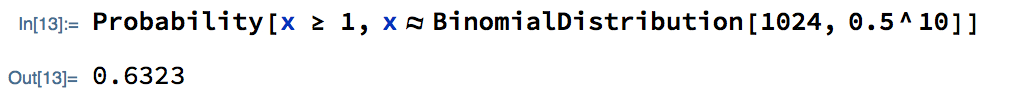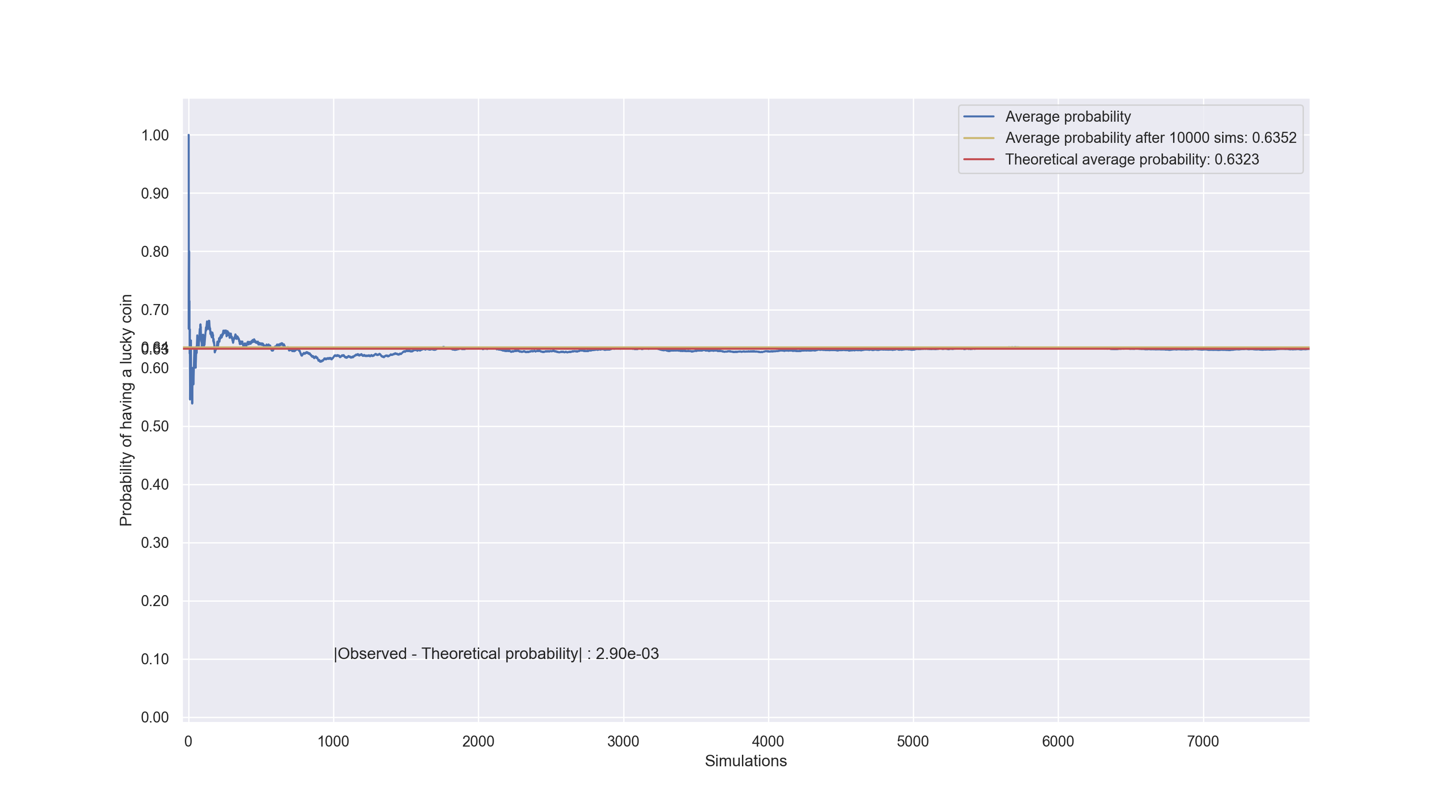I read this tweet today and it made me laugh out loud:

If 1024 fair coins are each tossed 10 times, chances are good (> 63%) that at least one will come up heads 10 times in a row; and that coin will be proud to explain how its skill, faith, guts & determination made its achievement possible, and how that combo can work for you too.

Maestro Nassim Nicholas Taleb has talked extensively about this phenomenon. In Fooled by Randomness he walks us through a thought experiment of 10000 fictional investment managers who each have 50% probability of making or losing \$10k at the end of the year. After 5 years, he concludes, 313 managers will make money, out of pure luck.

### Motivation

The extraordinary Incerto collection, my excitement about Machine Learning and my recent epiphany that most things around us are too complicated to understand, have all reinvigorated my interest in Probability & Statistics. I aim to keep training this muscle for years to come.

Using this witty coin-toss tweet as an opportunity, I will kickstart my math-related blog posts by computing the said probability (>63%) using a few different approaches.

### Analytical solution

#### Rule of Product

Let’s start with calculating the probability that a single coin that is tossed 10 times will come up heads 10 times.

Assumptions:

1. The consecutive coin tosses are independent events - the incidence of one event does not affect the probability of the other.
2. The coin is fair, which means that the probability that it will land on heads is $$0.5$$.

Based on (1) and (2) we can use the rule of product and simply calculate that:

$P(\underbrace{H, H, \ldots, H}_\text{10 times}) = \underbrace{0.5 \cdot 0.5 \cdot \ldots \cdot 0.5}_\text{10 times} = 0.5^{10}$

#### Binomial Distribution

Alternatively, we can model the process of tossing the coin 10 times as a random variable X that follows the binomial distribution ($$X \sim B(n,p)$$) where $$n=10$$ and $$p=\frac{1}{2}$$. The probability of getting exactly $$k$$ successes in $$n$$ independent trials with the chance of success being $$p$$, is given by the binomial probability mass function:

$P(X = k)=\binom{n}{k} p^k (1-p)^{n-k}$

For $$k = n = 10$$ we have:

$P(X = 10)=\binom{10}{10}0.5^{10}(1-0.5)^{0}=0.5^{10}$

which is equal to the result we got by using the rule of product.

#### Calculating the chances of that special coin

We found the probability that a coin tossed 10 times will come up heads every time. We now want to calculate the probability that at least one of 1024 tossed coins will land 10 consecutive heads. Like previously, we can model the 1024 coin tosses as a random variable $$Y$$, with $$Y \sim B(n,p)$$ where $$n=1024$$ and $$p=0.5^{10}$$. The probability we are looking for is:

$P(\small{\text{at least one of 1024 tossed coins lands 10 consecutive heads}})=\sum_{k=1}^{1024} P(Y=k)$

We have two ways to calculate this:

1. Compute the sum $\sum_{k=1}^{1024} P(Y=k)=\sum_{k=1}^{1024}\binom{1024}{k} (0.5^{10})^k (1-0.5^{10})^{1024-k}$
2. Knowing that:

\begin{align} \sum_{k=0}^{1024} P(Y=k) &= 1 \\ P(Y=0) + \sum_{k=1}^{1024} P(Y=k) &= 1 \\ \sum_{k=1}^{1024} P(Y=k) &= 1 - P(Y=0) \end{align}

then calculate $$1-P(Y=0)$$ instead. $$P(Y=0)$$ basically means that no coins came up with 10 consecutive heads. We will go with the second method:

\begin{align} P(\text{at least one of 1024 coins lands 10 consecutive heads}) &= 1 - P(Y=0) \\ &= 1 - \binom{1024}{0} (0.5^{10})^0 (1-(0.5^{10}))^{1024-0} \\ &= 1 - (1-0.5^{10})^{1024} \\ &= 1 - 0.367699 \\ &= 0.632301 \end{align}

So, as expected, we showed that the probability is > 63% .

## Mathematica

Mathematica is my go-to tool to build math intuition and to explore new problems. It is simple, powerful and has a beautiful interface. I get dopamine highs as soon as I launch the app and start typing equations.

Using mathematica we can get to our solution using the BinomialDistribution and Probability functions:How beautiful is this?

The BinomialDistribution function takes two arguments:

• The number of trials
• The success probability of a single trial

For our problem, the number of trials is 1024 and the success probability equals to $$0.5 ^{10}$$ as discussed in the previous section.

## Monte Carlo Simulation

Monte Carlo simulators are very powerful tools. Without the need of mathematical formality, we can get to our solution by “brute force”. Taleb (I promise it’s the last time I mention him in this post) has praised Monte Carlo Mathematics in Fooled byRandomness.

For our thought experiment, I wrote one just from scratch, assuming no probability knowledge:After 10000 simulations, there is 63.52 chance that at least one out of 1024 fair coins that are tossed 10 times will come up heads 10 times in a row. This is a pretty good approximation to the result we got from the previous sections.

## Summary

Inspired from a smart tweet by @bayesiangirl, this post presented 3 approaches to answer a simple, yet interesting, probability question. I could go further down the rabbithole and use more frameworks or libraries but I will stop here for now.